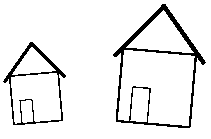Next: Plain TEX Up: iTe Previous: Invocation

# LATEX Commands

\usepackage[graphics|dvi]{ite}
Load the iTe package. Do not use \input ite.tex' unless your format is Plain TEX.

Example:

    \usepackage{ite}
The ite package can have one of the two following options:
graphics
This will use the rotation and scaling operations of the graphics or graphicx package. One of these packages is required. The dvi code produced is more device independent than without this option.
dvi.tex
Does not produce any device dependent code. No rotation and no scaling takes place.
Do not use these options for interactive use of iTe.
\usepackage{trig}
You have to load the trig package whenever you do not give dimensional arguments to an iteblock environment, see below.
\begin{iteblock}(x_dimen,y_dimen)
...
\end{iteblock}
The iteblock environment creates a box with the specified dimensions (optional) and serves as a container for \ite statements.

Example:

    \begin{iteblock}(\textwidth,5.0cm)
\ite Hallo
\end{iteblock}

The iteblock environment can contain any number of \ite statements. If the optional dimensional arguments are not given, the box will be the bounding box for all contained \ite objects. With the optional arguments, the box is framed for convenience. The use of nested, unrestricted iteblock environments is not allowed, see the definition of the itebind environment.

\ite ... 
Defines iTe object.

Example:

    \ite $cos x$
\ite \begin{minipage}Hello\\ World\end{minipage}

Whatever you can put inside an \mbox you can also put after an \ite statement. However, only restricted iteblock environments are allowed. iTe will expand each \ite statement to \ITE(x y a s), where x and y is the translation in units of \ITEunit, a is the angle of rotation, and s is the scaling factor of the object. You should not change these numbers manually.

\setlength{\ITEunit}{dimension}
The smallest unit an iTe object can be translated in either direction.

Example:

    \setlength{\ITEunit}{0.1pt}
The default is 0.5pt or approximately 0.35mm. You should change the value of \ITEunit only if a higher resolution is required. With the default, the two 'X' characters in the example
\ITE(0 0 0 1)X
\ITE(200 0 0 1)X
are 100pt apart. After \setlength{\ITEunit}{1mm}, the distance would be 200mm.
\begin{itebind}
...
\end{itebind}
The itebind environment deactivates all enclosed iTe objects. You have to use it inside macros, saved boxes, and recursive iteblock environments.

Example:
 \newsavebox{\housebox} \sbox{\housebox}{\begin{itebind} \begin{iteblock}(0cm,0cm) \ITE(0 0 0 1)\framebox(20,20){} \ITE(7 0 0 0.482)\framebox(10,20){} \ITE(19 66 -50 1)\rule{20pt}{1pt} \ITE(-4 35 50 1)\rule{20pt}{1pt} \end{iteblock} \end{itebind}} \begin{iteblock}(3cm,3cm) \ITE(87 95 5 1.728)\usebox{\housebox} \ITE(230 103 -5 2.589)\usebox{\housebox} \end{iteblock}`